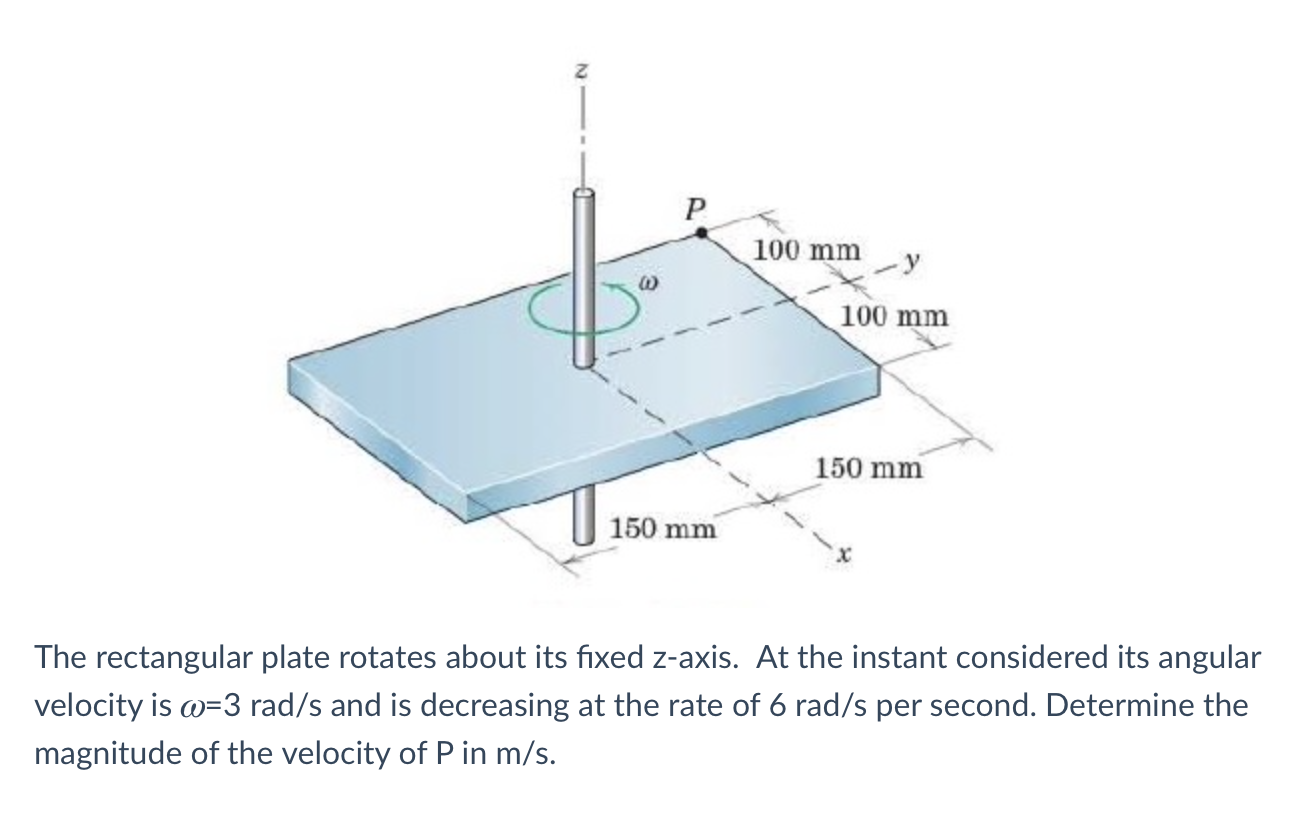# Question Z P 100 mm 100 mm 150 mm 150 mm The rectangular plate rotates about its fixed z-axis. At the instant considered its angular velocity is o=3 rad/s and is decreasing at the rate of 6 rad/s per second. Determine the magnitude of the velocity of P in m/s.XWLHVS The Asker · Mechanical EngineeringTranscribed Image Text: Z P 100 mm 100 mm 150 mm 150 mm The rectangular plate rotates about its fixed z-axis. At the instant considered its angular velocity is o=3 rad/s and is decreasing at the rate of 6 rad/s per second. Determine the magnitude of the velocity of P in m/s.
More
Transcribed Image Text: Z P 100 mm 100 mm 150 mm 150 mm The rectangular plate rotates about its fixed z-axis. At the instant considered its angular velocity is o=3 rad/s and is decreasing at the rate of 6 rad/s per second. Determine the magnitude of the velocity of P in m/s.